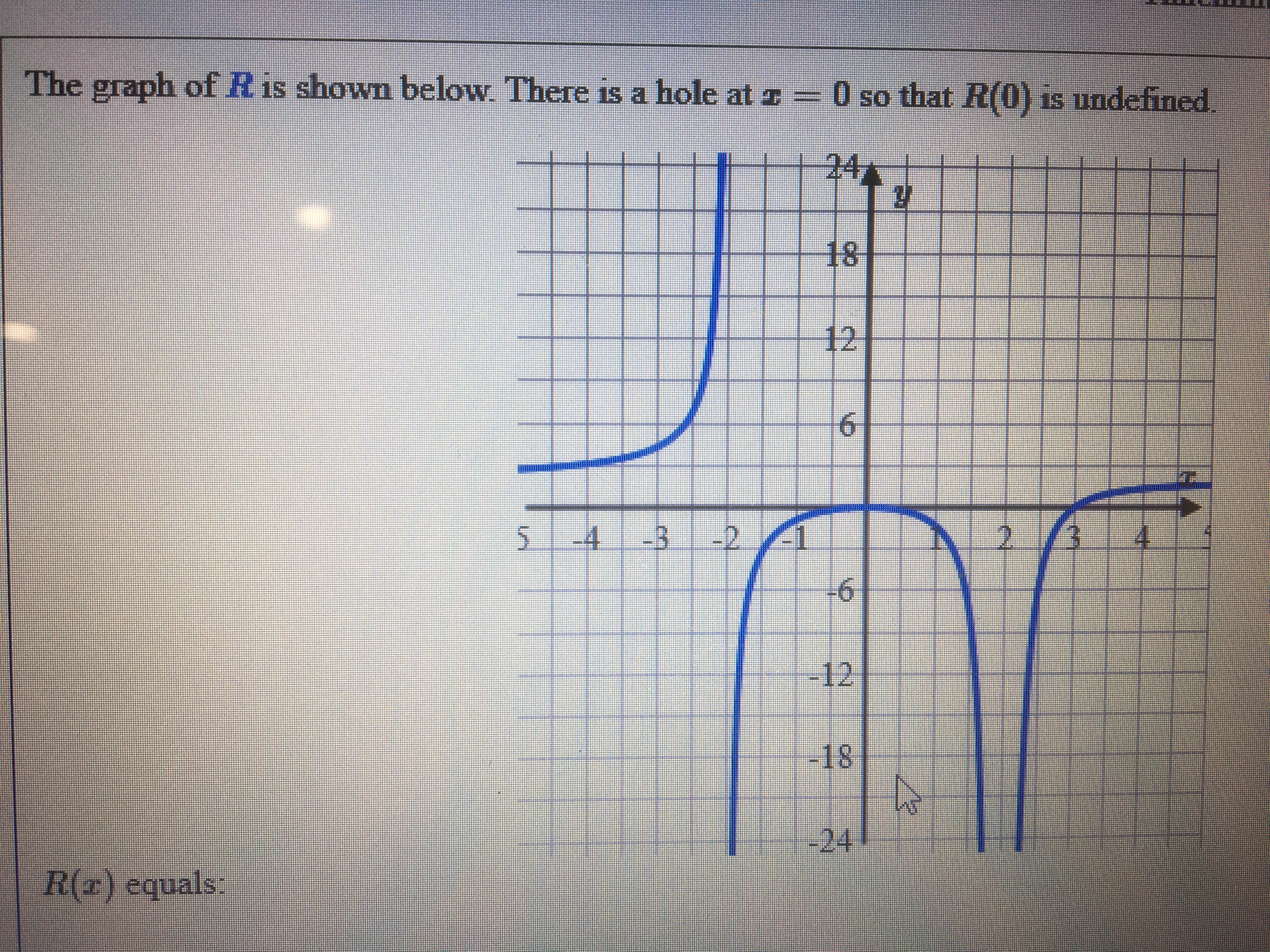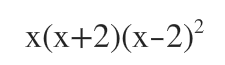# The graph of R is shown below. There is a hole at I = O so that R(0) is undefined2418126.-4.-B-22.-12-181.-24R(z) equals.

Question
6 views

What does r(x) equal?help_outlineImage TranscriptioncloseThe graph of R is shown below. There is a hole at I = O so that R(0) is undefined 24 18 12 6. -4. -B -2 2. -12 -18 1. -24 R(z) equals. fullscreen
check_circle

Step 1

R(x) has vertical asymptote at x=-2 and x=2

At x=2 degree is even. Because on both sides of x=2 the graph is going to the same side.

There is a hole at x=0

So, x will be a factor of both numerator and denominator

So the denominator will be...

### Want to see the full answer?

See Solution

#### Want to see this answer and more?

Solutions are written by subject experts who are available 24/7. Questions are typically answered within 1 hour.*

See Solution
*Response times may vary by subject and question.
Tagged in